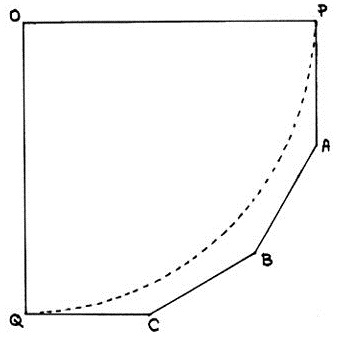# »  Solutions to puzzles in my National Review Online Diary

##September 2014

—————————

In my September 25th, 2014 VDARE column I posed the following brain-teaser.

My house has a small kitchen. The kitchen contains a table in one corner — that is, abutting two walls at right angles.

Since moving in 22 years ago we have lived with the previous owner's kitchen table in that spot. This table is circular, so for 22 years stuff has been falling off the table down the corner gap and we have had to crawl under the table to retrieve it, or assign a junior member of the family so to crawl.We have decided to get a new table for that corner. A square table is the obvious solution; but the kitchen is so small that the jutting-out corner (i.e. the one opposite the walls-corner) would be in the way, and an invitation to bang one's thigh against it when in a hurry.

How about a quadrant, then — a quarter-circle shape? That's OK, but they are surprisingly hard to find, and esthetically dull.

We have two kids, both now away from home; but on the occasions they are both with us, we want to sit and eat with them at the kitchen table, the family of four together.

My solution: A custom-made table of hexagonal shape, with two long sides OP, OQ at right angles (for the corner) and four shorter sides PA, AB, BC, CQ for us to sit at.

I know a guy who makes furniture; I'm going to give him the specs and ask for a quote.

Conditions: The two long sides are of equal length; the four short sides are all of equal length; the angles at O, P, and Q are right angles, and the angles at A, B, and C are all equal.

Questions: If the long sides are 40 inches, how long are the short sides? What are the lengths of OA and OB? What is the surface area of this table? How much bigger is it than an OPQ circular quadrant (dotted line)?

And: How many legs should this table have?

—————————

Solution

First let's simplify the scale: Set the long sides OP and OQ both equal to 1, and denote the short side by x.

The interior angles of a hexagon add to 720°. Since the angles at O, P, and Q are each 90°, while those at A, B, and C are all equal, each of the latter is 150°.

So we can extend the tabletop to a complete 1×1 square by adding two 30°-60°-90° triangles and a smaller square.The solutions now follow from elementary trigonometry, e.g. the properties of the 30°-60°-90° triangle. Since the hypoteneuse of each triangle is x by definition, the long sides are each x3/2 while the short sides (which are also the sides of the small square) are x/2.

Adding the three lengths along the bottom of the square:

x + x3/2 + x/2 = 1

We can solve that for x:

x = PA = AB = BC = 1 − √3/3

Basic algebra gives the rest:

OB = √2 − x/√2
OC² = OA² = x² + 1

For the area, just subtract the constructed triangles and square from the unit square:

Area = 1 − x²/4 − x²√3/4

That area comes out to (7 − √3)/6, which is about 11.79 percent bigger than the unit quadrant area of π/4.

In actual numbers, to six decimal places:

x = PA = AB = BC = 0.422650
OA = OC = 1.085649
OB = 1.115355
Area = 0.877992

Scaling up and switching to carpenter's units, to the nearest sixteenth of an inch:

x = AB = BC = 16 and 7/8.
OA = OC = 43 and 7/16.
OB = 44 and 5/8.
Area = 1,405 square inches, i.e. 9¾ square feet.

By cranking up the side length to 40½ inches, which space will indeed allow, I can get an even ten square feet of table area, compared with less than nine for the corresponding quadrant. (The other lengths of course need to be adjusted accordingly.)

Readers' suggestions for the number of legs range from one to six, with mode four.